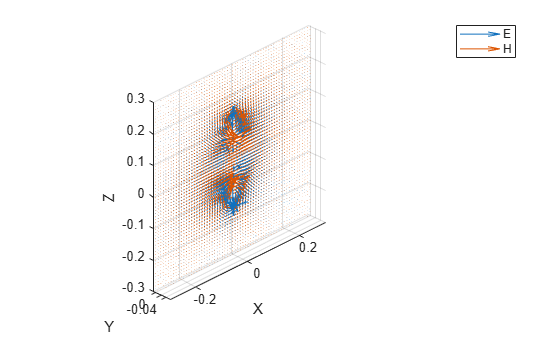Documentation

### This is machine translation

Mouseover text to see original. Click the button below to return to the English version of the page.

## Custom Radiation Pattern and Fields

This example shows how to visualize a radiation pattern and vector fields from user data. To plot 3D field data, use the `patternCustom` function. This function also allows the user to slice the data and see it. To visualize just 2D polar data use the `polarpattern` function. The `polarpattern` function allows you to interact with the data as well as perform antenna specific measurements. The user can also plot the vector fields at a point in space using the `fieldsCustom` function.

### Import 3D Pattern Data

3D Radiation pattern data stored in a csv file format can be read using the csvread function. In the first part of this example we use the patternCustom function to visualize the 3D data. The function can be used to visualize 2D slices of the 3D data as well.

`M = csvread('CustomPattern_testfile.csv',1,0);`

### Plot 3D Radiation Pattern on Polar Coordinate System

To plot the 3D radiation pattern on a polar coordinate system, specify the MagE vector/matrix and theta and phi vectors. If MagE is a matrix, it should be of size phi x theta. If MagE is a vector, all 3 arguments MagE, phi and theta should be of the same size.

`patternCustom(M(:,3),M(:,2),M(:,1));`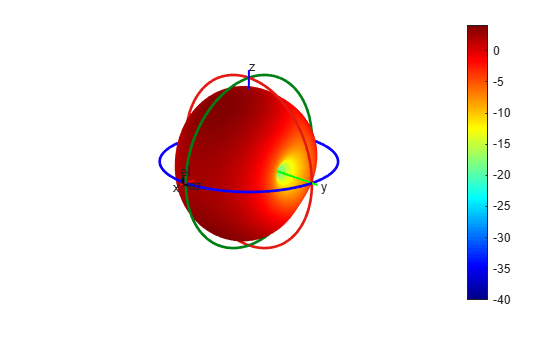### Plot 3D Radiation Pattern on Rectangular Coordinate System

To plot the 3D radiation pattern on a rectangular coordinate system, you modify the CoordinateSystem flag. By default, the flag is set to polar. Change it to rectangular to visualize the data in the rectangular coordinate system.

`patternCustom(M(:,3),M(:,2),M(:,1),'CoordinateSystem','rectangular');`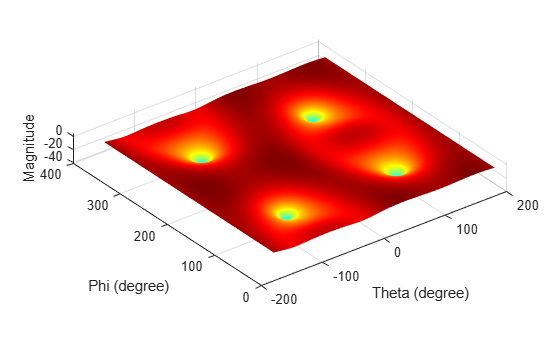### Visualize 2D Slices from the 3D Data

To plot a 2D slice on polar coordinate system, modify the Slice flag to either 'phi' or 'theta', depending on the plane you want to view data in. You should also modify the SliceValue flag to give a vector of phi or theta values for the slices. The slice values should be in the input data. Specify the CoordinateSystem flag as polar to view using a polar plot.

```patternCustom(M(:,3),M(:,2),M(:,1),'CoordinateSystem','polar','Slice', ... 'phi','SliceValue',[45 90 180 360]);```Specify the CoordinateSystem flag as rectangular to view the above case using a rectangular plot.

```patternCustom(M(:,3),M(:,2),M(:,1),'CoordinateSystem','rectangular', ... 'Slice','phi','SliceValue',[45 90 180 360]);```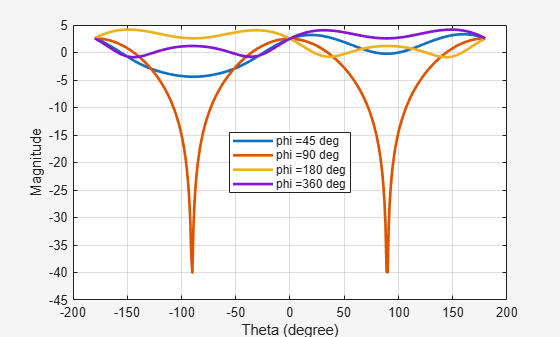### Plot 2D Polar Data

To plot a 2D polar data, you can use the `polarpattern` function as shown below. The plot generated is an interactive plot that allows the user to perform antenna specific measurements as well. The data in this case is stored in a .mat file. The file contains directivity values calculated over 360 degrees with one degree separation.

```load polardata p = polarpattern(ang, D);```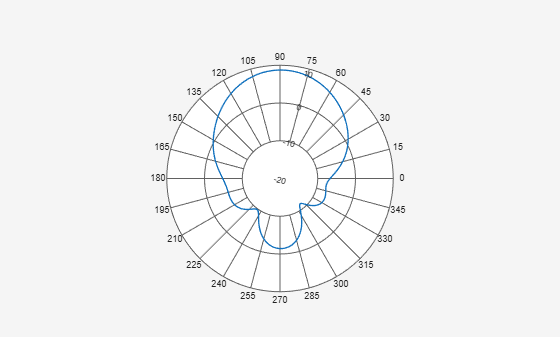Right click in the figure window to interact with the plot. The figure below shows a screen shot of the context menu. The context menus can be used to do measurements such as peak detection, beamwidth calculation etc. You can also add a cursor by right clicking inside the polar circle.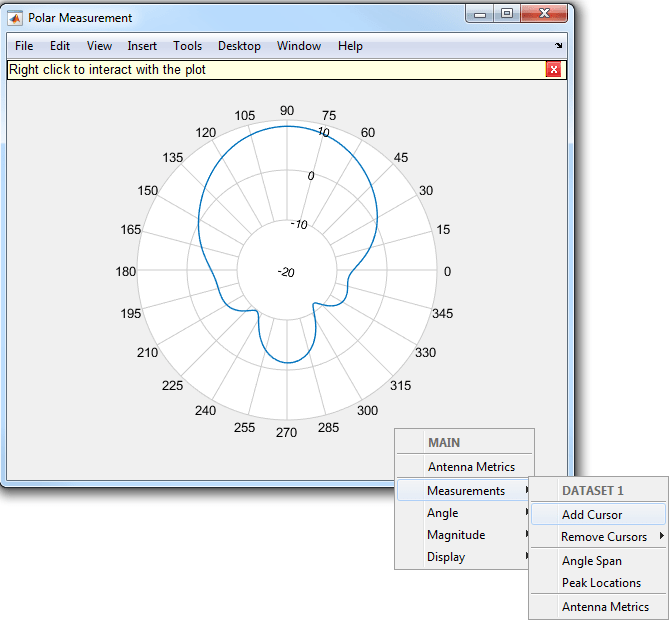Select the Antenna Metrics option in the context menu shown above, to visualize the antenna specific measurements as shown below.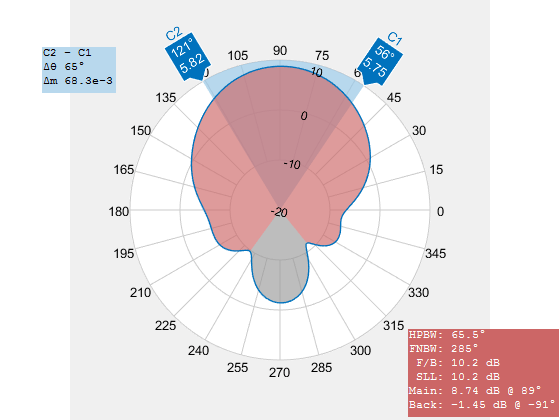### Plot Vector Field Data at a Point in Space

To plot vector electric and/or magnetic fields at any point in space, use the `fieldsCustom` function as shown below. The mat file EHfielddata contains the E and H field data as well as the points in space specified as x, y and z coordinates. The electric and magnetic fields are complex quantities and have x, y and z components at every point in space. The fields can be artificially scaled for better visualization.

```load EHfielddata; figure; fieldsCustom(H, points, 5);```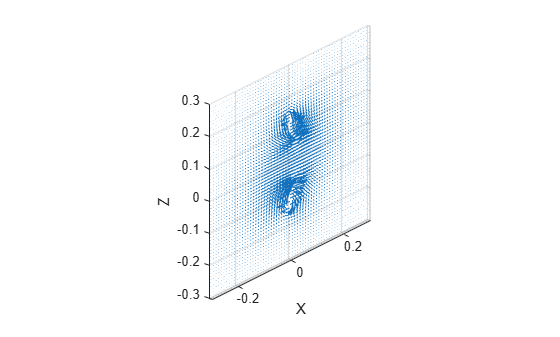The function is used to plot one field quantity at a time. To plot both E and H fields on the same plot, use the hold on command.

```figure; fieldsCustom(gca, E, points, 5); hold on; fieldsCustom(gca, H, points, 5); hold off; legend('E', 'H');```